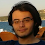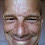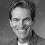## Wednesday, December 27, 2017

### The Fiscal Theory of Monetary Policy

"Stepping on a Rake: the Fiscal Theory of Monetary Policy" is new paper, just published in the European Economic Review. This link gets you free access, but just for the next few days. After that, I can only post the last manuscript. (I held off sending this hoping the EER would fix the figure placement in the html version, but that didn't happen.)

The paper is about how the fiscal theory of the price level can describe monetary policy. Even without monetary, pricing, or financial frictions, the central bank can fix interest rates. In the presence of long-term debt higher interest rates lead to lower inflation for a while. Interest rate targets, forward guidance, and quantitative easing all work by the same mechanism. The paper also derives Chris Sims' "stepping on a rake" paper which makes that point, and integrates fiscal theory with a detailed new Keynesian model in continuous time.

1."The standard intuition that higher interest rates lead to lower inflation comes from adaptive expectations models. In these models, the Fisher equation is an unstable steady state, so a persistent rise in the nominal rate sends inflation spiraling off negatively. Real rates rises by more than the nominal rate. Adaptive expectations thinking remains strong in policy circles, so the short run negative sign seems natural and the long run positive sign seems puzzling in that context. But current economic theory in forward-looking models goes the opposite way."

If we follow the NK Taylor rule approach and rule out explosive paths, then adaptive expectations models (like the one Friedman used to show that nominal interest rate pegs are unstable) are as "stable" as rational expectations models.

In addition, if you take the New Keynesian model and add adaptive expectations to it, say in the form of dx/dt = (i - R - pi^e)/s, d pi^e/dt = b(pi - pi^e), x = k(pi - pi^e); you get a system which has two imaginary eigenvalues under a nominal interest rate peg, so it spirals around the steady state. It's not even unstable in the traditional sense of the word, i.e positive eigenvalues, and it is stabilized by a policy rule such as i = R + pi* - cx for some c > 0, which goes in the face of conventional thinking about monetary policy.

The "dynamic IS curve" of modern macro is just very different from the static IS curve of IS-LM, and the reason people get confused about this is because they are following Krugman's advice of thinking in terms of IS-LM and only using New Keynesian models when you need to write a paper. I think we need to speak as loudly as we can about this point so that people stop doing this. The problem isn't adaptive expectations, it's static vs dynamic models.

2.Suppose we had a Central Bank that outright refused to monetize government deficits (I think Sargent and Wallace labeled this as a "ricardian regime"), would the fiscal theory of the price level be useful in explaining inflation past, present and future?

I would say that the US fits this sort of regime. FTPL might be useful in examining Venezuela/Zimbabwe but how does this help us explain things better
than the modern quantity theory?

1.Not without accounting for total debt outstanding (public and private) or productivity (real GDP / total debt).

Higher stable nominal and real interest rates are possible if they are supported by higher productivity.

3.Does this mean the Bank of Japan should keep rates where they are, near zero?

4.The Fischer effect is the designated equilibrium point. We should always see it if you can look back after all prices are collected.

The presence of long term debt means your government is vulnerable to term premium risk, so we introduce some term risk with an arbitrary interest change, and we see the system redistribute the discovered risk and return to Fischer.

It is the fiscal theory of price, but short term effects are driven by the illiquidity of government.

5.This comment has been removed by the author.

6.John,

FTPL is incomplete because it does not address productivity, changes in total debt (public and private), or changes in GDP.

NGDP = P * Q
dNGDP / dt = Q * dP/dt + P * dQ/dt
dNGDP = Q * dP + P * dQ
dP / P = dNGDP / NGDP - dQ / Q = dNGDP / NGDP - dRDGP / RGDP

-------- K = Productivity -----------
-------- TCMDO = Total Debt (Public and Private) -----------

K = RGDP / TCMDO
RGDP = K * TCMDO
dRGDP / dt = dK/dt * TCMDO + dTCMDO/dt * K
dRGDP / RGDP = dK/K + dTCMDO/TCMDO

Combining the two equations:
dP / P = dNGDP / NGDP - dK/K - dTCMDO/TCMDO

Fisher Equation
i(t) = dP / P + r(t)

i(t) = dNGDP / NGDP - dK/K - dTCMDO/TCMDO + r(t)

Solving for the real interest rate r(t):

r(t) = i(t) - dNGDP / NGDP + dK/K + dTCMDO/TCMDO

It should be apparent from this equation that a rise in the nominal interest rate i(t) will cause a permanent increase in the real interest rate r(t) as long as:

---- Correction -----
dK/K + dTCMDO/TCMDO - dNGDP / NGDP > 0
---------------------

If you want to offer a fiscal angle then start with this - what are the productivity effects of government borrowing?

Specifically, for the federal government, what is the value of K?

1.I don't get it. You defined K as real gdp / total debt. What does that have to do with productivity? Why is that a constant, not just the ratio of arbitrary numbers? FTPL starts with the valuation equation for government debt , value of government debt = expected present value of future surpluses that pay back the debt. Productivity shows up in the discount factor, and that higher productivity means higher GDP and hence higher surpluses.

2.This comment has been removed by the author.

3.John,

"You defined K as real gdp / total debt. What does that have to do with productivity?"

Productivity defined as real GDP / total debt has some advantages over measures like total factor productivity:

https://en.wikipedia.org/wiki/Total_factor_productivity

1. Productivity defined this way includes sectors where "widgets" are not created (government, banking, etc.).

2. Productivity defined this was has meaningful units of measure in widgets per dollar (unlike TFP).

3. Productivity defined this way can be (on the macro level) easily measured. See:
https://fred.stlouisfed.org/series/TCMDO
https://fred.stlouisfed.org/series/GDPC1

"Why is that a constant, not just the ratio of arbitrary numbers?"

See the equations above. I know that K is sometimes used to define a constant in economics, but I am using it as a variable here (notice the references to both dK/dt and dK/K).

"Productivity shows up in the discount factor, and that higher productivity means higher GDP and hence higher surpluses."

First, both in mine and in the Cobb Douglas equation, higher productivity does not guarantee higher real GDP. Productivity is measured as a ratio of outputs to inputs (for instance debt input to real GDP output).

In my formulation for productivity, even if productivity increases by 5%, real GDP output will fall if debt falls by 10%.

Second, productivity is not referenced once in the paper that you provide a link for:

http://faculty.chicagobooth.edu/john.cochrane/research/papers/sims_replication_elsarticle.pdf

And the only reference to a discount factor is in section #2.1 of your paper:

"Use a constant real discount factor"
"β = 1/(1 + r)"
"where r denotes the real rate of interest."

7.John,

Also, the only mention of a discount factor that I could find in your paper was this:

Section 2
"Use a constant real discount factor"
"β = 1/(1 + r)"
"where r denotes the real rate of interest"

I could find no other reference to either productivity or a discount factor.

1.Now I see. In academic articles we often use a simplifying assumption to keep the algebra clean, when it's easy to see that one could generalize the assumption and it would make no difference to the result. FTPL works with arbitrarily general discount factors.

2.John,

That doesn't make a lot of sense if we accept that productivity is time varying and is independent of other variables.

Comments are welcome. Keep it short, polite, and on topic.

Thanks to a few abusers I am now moderating comments. I welcome thoughtful disagreement. I will block comments with insulting or abusive language. I'm also blocking totally inane comments. Try to make some sense. I am much more likely to allow critical comments if you have the honesty and courage to use your real name.